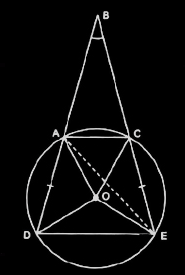Guru

# Let the vertex of an angle ABC be located outside a circle and let the sides of the angle intersect equal chords AD and CE with the circle. Prove that ∠ABC is equal to half the difference of the angles subtended by the chords AC and DE at the centre. Q.4

• 0

How can i solve this tough question of class 9th ncert of Circles of math of exercise 10.6 of question no.4 . Give me the best and simple way for solving this question. Let the vertex of an angle ABC be located outside a circle and let the sides of the angle intersect equal chords AD and CE with the circle. Prove that ∠ABC is equal to half the difference of the angles subtended by the chords AC and DE at the centre.

Share

1. Consider the diagramWe know, any exterior angle of a triangle is equal to the sum of interior opposite angles.

So,

∠DAE = ∠ABC+∠AEC (in ΔBAE) ——————-(i)

DE subtends ∠DOE at the centre and ∠DAE in the remaining part of the circle.

So,

∠DAE = (½)∠DOE ——————-(ii)

Similarly, ∠AEC = (½)∠AOC  ——————-(iii)

Now, from equation (i), (ii), and (iii) we get,

(½)∠DOE = ∠ABC+(½)∠AOC

Or, ∠ABC = (½)[∠DOE-∠AOC]  (hence proved).

• 0